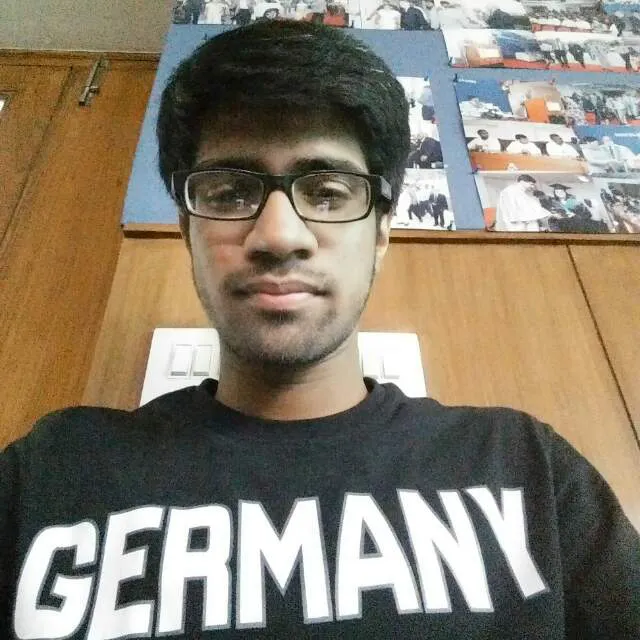Published

# Ball Tracking Robot

A robo which follows an object of uniform color using Computer Vision.

IntermediateShowcase (no instructions)72,711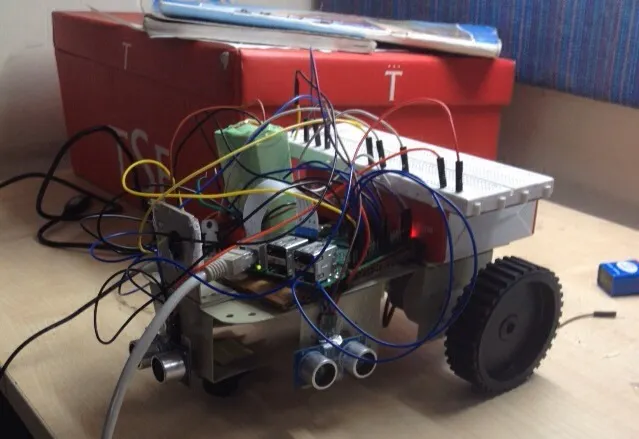## Things used in this project

### Hardware components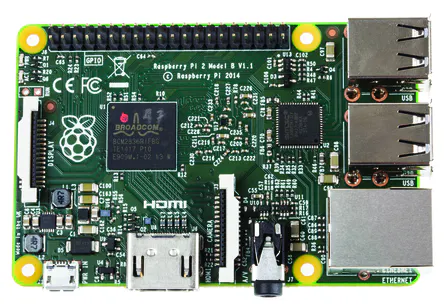Raspberry Pi 2 Model B
×1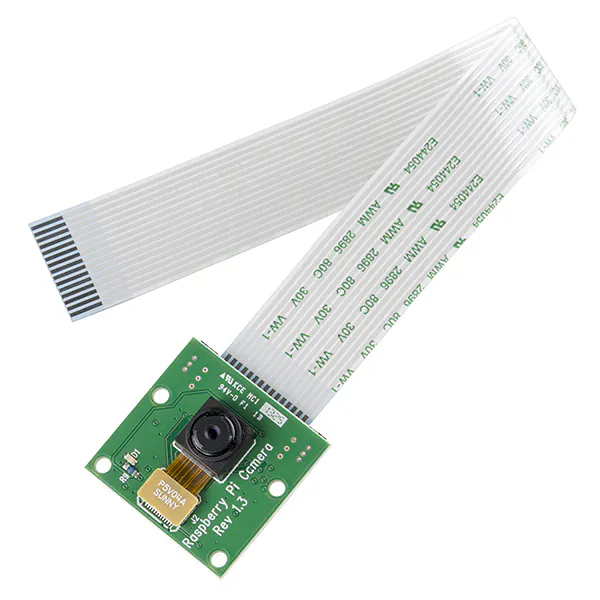Raspberry Pi Camera Module
×1
 Arduino Ultrasonic Sensor
×3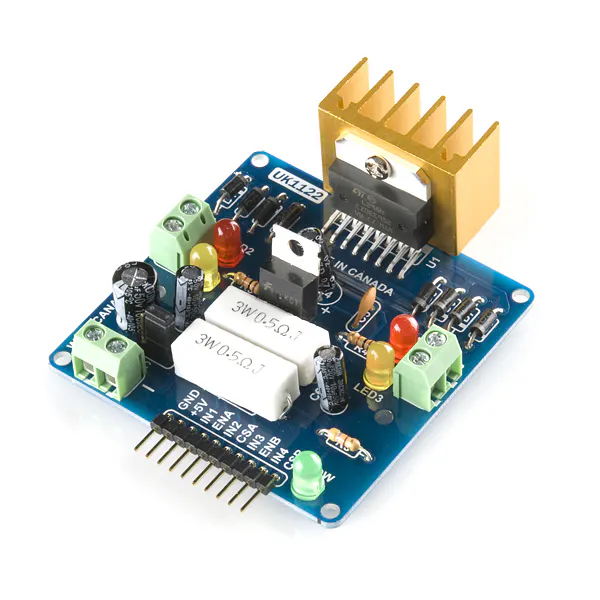SparkFun Dual H-Bridge motor drivers L298
×2
 DC motor (generic)
×2×1
 Connectors/ Wires
×1

### Software apps and online servicesOpenCV

 Python

## Schematics

### Circuit Diagram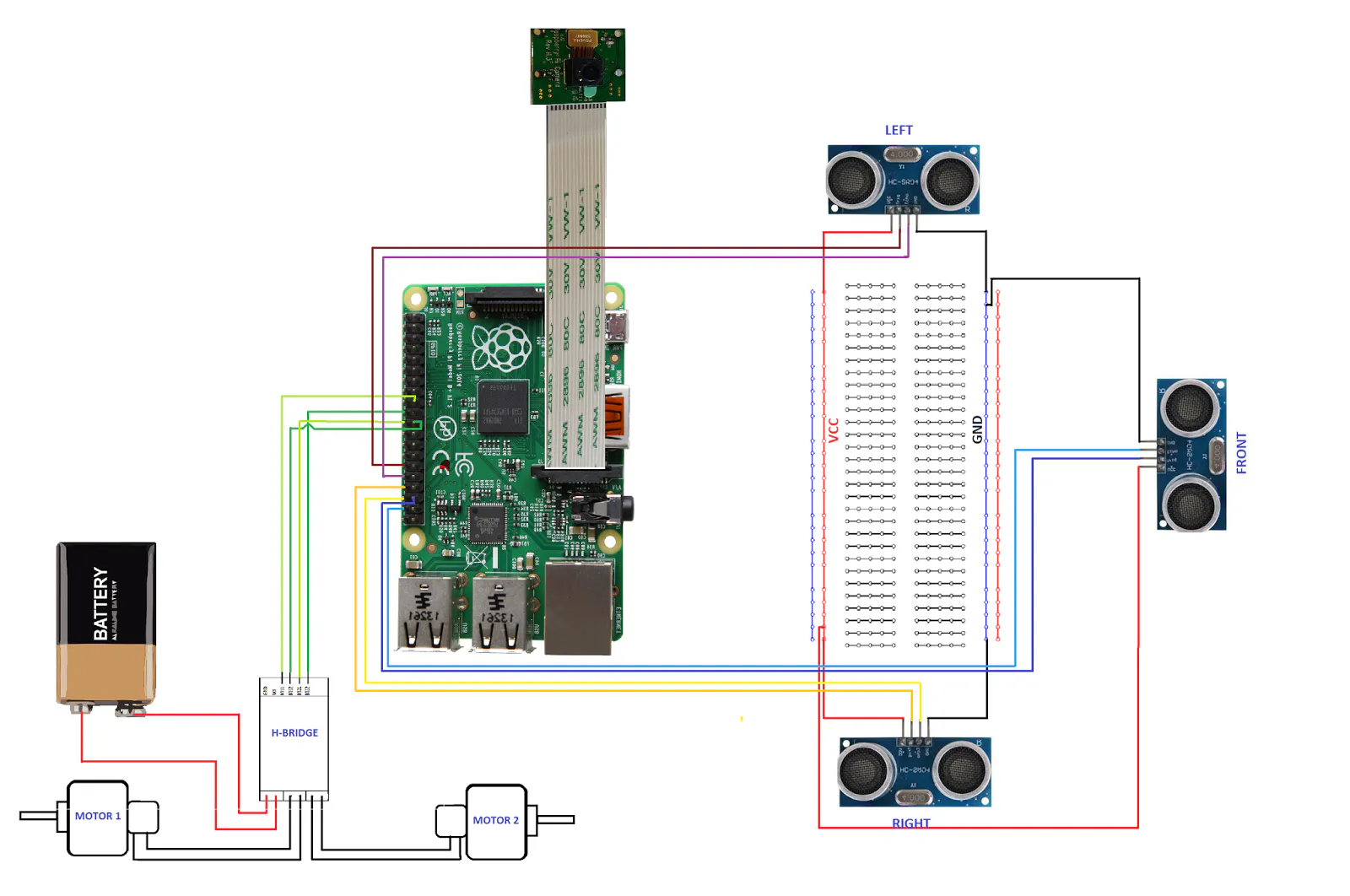### Pseudo code for Computer Vision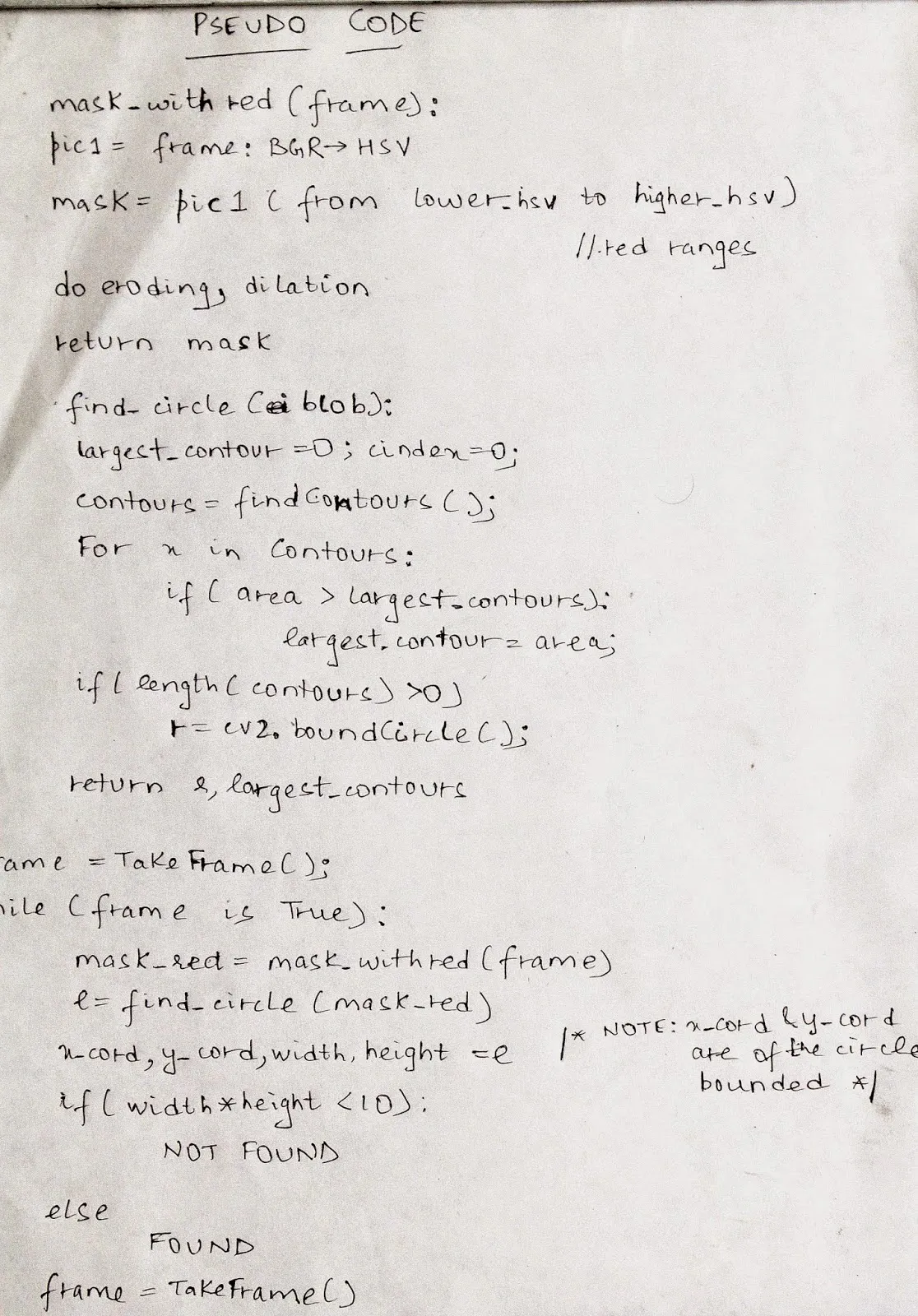### Circuit Diagram## Code

### Code

Python
Upload in your Raspberry Pi and make a shell script to run it as soon as Raspberry Pi starts.
```# import the necessary packages
from picamera.array import PiRGBArray     #As there is a resolution problem in raspberry pi, will not be able to capture frames by VideoCapture
from picamera import PiCamera
import RPi.GPIO as GPIO
import time
import cv2
import cv2.cv as cv
import numpy as np

#hardware work
GPIO.setmode(GPIO.BOARD)

GPIO_TRIGGER1 = 29      #Left ultrasonic sensor
GPIO_ECHO1 = 31

GPIO_TRIGGER2 = 36      #Front ultrasonic sensor
GPIO_ECHO2 = 37

GPIO_TRIGGER3 = 33      #Right ultrasonic sensor
GPIO_ECHO3 = 35

MOTOR1B=18  #Left Motor
MOTOR1E=22

MOTOR2B=21  #Right Motor
MOTOR2E=19

LED_PIN=13  #If it finds the ball, then it will light up the led

# Set pins as output and input
GPIO.setup(GPIO_TRIGGER1,GPIO.OUT)  # Trigger
GPIO.setup(GPIO_ECHO1,GPIO.IN)      # Echo
GPIO.setup(GPIO_TRIGGER2,GPIO.OUT)  # Trigger
GPIO.setup(GPIO_ECHO2,GPIO.IN)
GPIO.setup(GPIO_TRIGGER3,GPIO.OUT)  # Trigger
GPIO.setup(GPIO_ECHO3,GPIO.IN)
GPIO.setup(LED_PIN,GPIO.OUT)

# Set trigger to False (Low)
GPIO.output(GPIO_TRIGGER1, False)
GPIO.output(GPIO_TRIGGER2, False)
GPIO.output(GPIO_TRIGGER3, False)

# Allow module to settle
def sonar(GPIO_TRIGGER,GPIO_ECHO):
start=0
stop=0
# Set pins as output and input
GPIO.setup(GPIO_TRIGGER,GPIO.OUT)  # Trigger
GPIO.setup(GPIO_ECHO,GPIO.IN)      # Echo

# Set trigger to False (Low)
GPIO.output(GPIO_TRIGGER, False)

# Allow module to settle
time.sleep(0.01)

#while distance > 5:
#Send 10us pulse to trigger
GPIO.output(GPIO_TRIGGER, True)
time.sleep(0.00001)
GPIO.output(GPIO_TRIGGER, False)
begin = time.time()
while GPIO.input(GPIO_ECHO)==0 and time.time()<begin+0.05:
start = time.time()

while GPIO.input(GPIO_ECHO)==1 and time.time()<begin+0.1:
stop = time.time()

# Calculate pulse length
elapsed = stop-start
# Distance pulse travelled in that time is time
# multiplied by the speed of sound (cm/s)
distance = elapsed * 34000

# That was the distance there and back so halve the value
distance = distance / 2

print "Distance : %.1f" % distance
# Reset GPIO settings
return distance

GPIO.setup(MOTOR1B, GPIO.OUT)
GPIO.setup(MOTOR1E, GPIO.OUT)

GPIO.setup(MOTOR2B, GPIO.OUT)
GPIO.setup(MOTOR2E, GPIO.OUT)

def forward():
GPIO.output(MOTOR1B, GPIO.HIGH)
GPIO.output(MOTOR1E, GPIO.LOW)
GPIO.output(MOTOR2B, GPIO.HIGH)
GPIO.output(MOTOR2E, GPIO.LOW)

def reverse():
GPIO.output(MOTOR1B, GPIO.LOW)
GPIO.output(MOTOR1E, GPIO.HIGH)
GPIO.output(MOTOR2B, GPIO.LOW)
GPIO.output(MOTOR2E, GPIO.HIGH)

def rightturn():
GPIO.output(MOTOR1B,GPIO.LOW)
GPIO.output(MOTOR1E,GPIO.HIGH)
GPIO.output(MOTOR2B,GPIO.HIGH)
GPIO.output(MOTOR2E,GPIO.LOW)

def leftturn():
GPIO.output(MOTOR1B,GPIO.HIGH)
GPIO.output(MOTOR1E,GPIO.LOW)
GPIO.output(MOTOR2B,GPIO.LOW)
GPIO.output(MOTOR2E,GPIO.HIGH)

def stop():
GPIO.output(MOTOR1E,GPIO.LOW)
GPIO.output(MOTOR1B,GPIO.LOW)
GPIO.output(MOTOR2E,GPIO.LOW)
GPIO.output(MOTOR2B,GPIO.LOW)

#Image analysis work
def segment_colour(frame):    #returns only the red colors in the frame
hsv_roi =  cv2.cvtColor(frame, cv2.cv.CV_BGR2HSV)
mask_1 = cv2.inRange(hsv_roi, np.array([160, 160,10]), np.array([190,255,255]))
ycr_roi=cv2.cvtColor(frame,cv2.cv.CV_BGR2YCrCb)

kern_dilate = np.ones((8,8),np.uint8)
kern_erode  = np.ones((3,3),np.uint8)

def find_blob(blob): #returns the red colored circle
largest_contour=0
cont_index=0
contours, hierarchy = cv2.findContours(blob, cv2.RETR_CCOMP, cv2.CHAIN_APPROX_SIMPLE)
for idx, contour in enumerate(contours):
area=cv2.contourArea(contour)
if (area >largest_contour) :
largest_contour=area

cont_index=idx
#if res>15 and res<18:
#    cont_index=idx

r=(0,0,2,2)
if len(contours) > 0:
r = cv2.boundingRect(contours[cont_index])

return r,largest_contour

def target_hist(frame):
hsv_img=cv2.cvtColor(frame, cv2.COLOR_BGR2HSV)

hist=cv2.calcHist([hsv_img],,None,,[0,255])
return hist

#CAMERA CAPTURE
#initialize the camera and grab a reference to the raw camera capture
camera = PiCamera()
camera.resolution = (160, 120)
camera.framerate = 16
rawCapture = PiRGBArray(camera, size=(160, 120))

# allow the camera to warmup
time.sleep(0.001)

# capture frames from the camera
for image in camera.capture_continuous(rawCapture, format="bgr", use_video_port=True):
#grab the raw NumPy array representing the image, then initialize the timestamp and occupied/unoccupied text
frame = image.array
frame=cv2.flip(frame,1)
global centre_x
global centre_y
centre_x=0.
centre_y=0.
hsv1 = cv2.cvtColor(frame, cv2.COLOR_BGR2HSV)
x,y,w,h=loct

#distance coming from front ultrasonic sensor
distanceC = sonar(GPIO_TRIGGER2,GPIO_ECHO2)
#distance coming from right ultrasonic sensor
distanceR = sonar(GPIO_TRIGGER3,GPIO_ECHO3)
#distance coming from left ultrasonic sensor
distanceL = sonar(GPIO_TRIGGER1,GPIO_ECHO1)

if (w*h) < 10:
found=0
else:
found=1
simg2 = cv2.rectangle(frame, (x,y), (x+w,y+h), 255,2)
centre_x=x+((w)/2)
centre_y=y+((h)/2)
cv2.circle(frame,(int(centre_x),int(centre_y)),3,(0,110,255),-1)
centre_x-=80
centre_y=6--centre_y
print centre_x,centre_y
initial=400
flag=0
GPIO.output(LED_PIN,GPIO.LOW)
if(found==0):
#if the ball is not found and the last time it sees ball in which direction, it will start to rotate in that direction
if flag==0:
rightturn()
time.sleep(0.05)
else:
leftturn()
time.sleep(0.05)
stop()
time.sleep(0.0125)

elif(found==1):
if(area<initial):
if(distanceC<10):
#if ball is too far but it detects something in front of it,then it avoid it and reaches the ball.
if distanceR>=8:
rightturn()
time.sleep(0.00625)
stop()
time.sleep(0.0125)
forward()
time.sleep(0.00625)
stop()
time.sleep(0.0125)
#while found==0:
leftturn()
time.sleep(0.00625)
elif distanceL>=8:
leftturn()
time.sleep(0.00625)
stop()
time.sleep(0.0125)
forward()
time.sleep(0.00625)
stop()
time.sleep(0.0125)
rightturn()
time.sleep(0.00625)
stop()
time.sleep(0.0125)
else:
stop()
time.sleep(0.01)
else:
#otherwise it move forward
forward()
time.sleep(0.00625)
elif(area>=initial):
initial2=6700
if(area<initial2):
if(distanceC>10):
#it brings coordinates of ball to center of camera's imaginary axis.
if(centre_x<=-20 or centre_x>=20):
if(centre_x<0):
flag=0
rightturn()
time.sleep(0.025)
elif(centre_x>0):
flag=1
leftturn()
time.sleep(0.025)
forward()
time.sleep(0.00003125)
stop()
time.sleep(0.00625)
else:
stop()
time.sleep(0.01)

else:
#if it founds the ball and it is too close it lights up the led.
GPIO.output(LED_PIN,GPIO.HIGH)
time.sleep(0.1)
stop()
time.sleep(0.1)
#cv2.imshow("draw",frame)
rawCapture.truncate(0)  # clear the stream in preparation for the next frame

if(cv2.waitKey(1) & 0xff == ord('q')):
break

GPIO.cleanup() #free all the GPIO pins
```

## Credits

### Rohan Juneja

1 project • 53 followers
I am a student of IIITD, Delhi, India majoring in Electronics and Communication Engineering. Keen intersts: Computer Architecture, Embedded Systems and IoT.